# Test: Equations Reducible to Linear Form

## 20 Questions MCQ Test NCERT Mathematics for CAT Preparation | Test: Equations Reducible to Linear Form

Description
Attempt Test: Equations Reducible to Linear Form | 20 questions in 20 minutes | Mock test for Class 10 preparation | Free important questions MCQ to study NCERT Mathematics for CAT Preparation for Class 10 Exam | Download free PDF with solutions
QUESTION: 1

Solution:
QUESTION: 2

Solution:
QUESTION: 3

### The sum of ages of A and B is 49 years. A said to B, “I am twice as old as you were when I was as old as you are. Find the present age of B.

Solution:

Let the ages of A and B be a and b
Going by the statement step by step
A says  “I am twice as old”=A says that age is twice some number which is a=2( _ )
"as you were" which talks about past, means B’s age some times ago...which is b-(how many years ago)
So, a=2(b- (how many years ago))
“when I was as old as you are" = when A was B’s age now= When A was b years old. So no. of years from now to back then will be A’s age now minus A’s age then =a-b
a=2(b-(a-b))
a=4b-2a
a=4b/3    …..1
Also a+b=49
a=49-b      …..2
From 1 and 2
49-b=4b/3
4b/3+b=49
7b/3=49
b=49*3/7=21 years
a=28 years

QUESTION: 4

The value of x, y in the following pair of Linear equations is: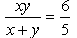,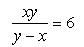Solution:
QUESTION: 5

The value ofx,y in the following pair of Linear equations is: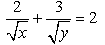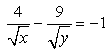Solution: Firstly, let 1/√x= a and 1/√y=b
=> 2a+3b=2   -(1)
=> 4a-9b= -1 -(2)
Now,  adding 3 times if equation (1)  with equation (2)
=>    6a+9b=6
4a-9b=-1
=>   10a=5
=> a=1/2
Now, Subsituting the value of a in equation (1)
2(1/2) +3b=2
1+3b=2
3b=2-1
b=-1/3
Now, as a=1/√x and b=1/√y
1/√x= 1/2 and 1/√y=1/3
=> √x=2 and √y=3
Squaring both sides
=> x=4 and y=9
QUESTION: 6

The value of x, y in the following 2x + 2 – 3y + 1 = 5 ………(1) , 2x + 3y = 17 ………(2)​

Solution:
QUESTION: 7

The value of x, y in the following pair of Linear equations is: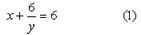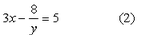Solution:
QUESTION: 8

The value of x, y in the following pair of Linear equations is: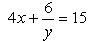,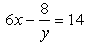Solution:
QUESTION: 9

The value ofx, y in the following pair of Linear equations is: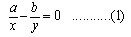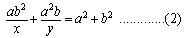Solution:
QUESTION: 10

If 6x + 3y = 6xy; 2x + 4y = 5xy, then the values of x and y are​

Solution:
QUESTION: 11

The values of x and y , if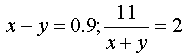Solution:
QUESTION: 12

A boat goes 35 km upstream and 42 km downstream in 11 hours. In 14 hours, it can go 43 km upstream and 59 km down-stream. To determine the speed of the stream and that of the boat in still water , express the information in the form of equations of the type ax + by + c = 0

Solution:
QUESTION: 13

In an examination, one mark is awarded for every correct answer, while 1/4 mark is deducted for every wrong answer. A student answered 120 questions and got 20 marks. Which of the following pair of equations would give the result for how many questions did he answered correctly?​

Solution:
QUESTION: 14

The value of x, y in the following pair of Linear equations is: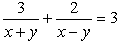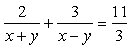Where  x + y ≠ 0 and x - y ≠ 0 ?

Solution:
QUESTION: 15

The solution of the following pair of Linear equations is: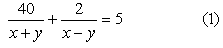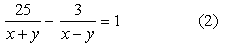Solution:
QUESTION: 16

Find x and y given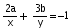,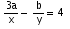Solution:
QUESTION: 17

3 women and 6 men can together finish a tailoring job in 5 days, while 4 women and 7 men can finish it in 4 days. Find the time taken by 1 woman alone to finish the work, and also that taken by 1 man alone. The linear equations(in standard form) to solve this problem algebraically are​

Solution:
QUESTION: 18

The values of x and y that satisfy the pair of equations: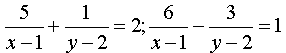Solution:
QUESTION: 19

If 6x + 3y= 6xy ; 2x + 4y = 5xy, then the values of xand y are​

Solution:
QUESTION: 20

By selling a wrist watch of Rs.405 the shopkeeper incurs a loss of 10%. What is the gain or loss percentage if he sells the same watch at Rs. 465?

Solution:

CP = 405 / 0.9 = 450
New SP = Rs. 465
Profit (%) = [465-450 / 450] X 100 = 3.33%Use Code STAYHOME200 and get INR 200 additional OFF Use Coupon Code